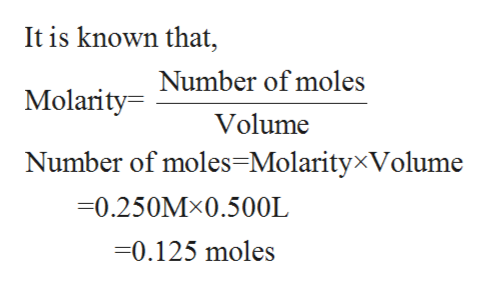What volume of 0.250 M lithium hydroxide will completely react with 0.500 L of 0.250 M of sulfuric acid solution?The website says that the answer is 0.250 LiOH but I keep getting 0.5 LiOH

Question

What volume of 0.250 M lithium hydroxide will completely react with 0.500 L of 0.250 M of sulfuric acid solution?

The website says that the answer is 0.250 LiOH but I keep getting 0.5 LiOH

Step 1

The term molarity has been defined as the ratio of number of moles of a substance to the volume of the give n solution in liters. Its SI units are moles/liter, which can also be denoted as ‘M’.

Step 2

The chemical reaction which takes place between sulfuric acid and lithium hydroxide is shown below in the balanced chemical equation:

Step 3

The number of moles of H2SO4 are calcula...help_outlineImage TranscriptioncloseIt is known that, MolarityNumber of moles Volume Number of moles=MolarityxVolume -0.250MX0.500L -0.125 moles fullscreen

Want to see the full answer?

See Solution

Want to see this answer and more?

Our solutions are written by experts, many with advanced degrees, and available 24/7

See Solution
Tagged in

Inorganic Chemistry